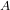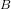## You exert a 138 N push the leftmost of two identical blocks of mass 244 g connected by a spring of stiffness 605 kg/s2. After pushing the bl

Question

You exert a 138 N push the leftmost of two identical blocks of mass 244 g connected by a spring of stiffness 605 kg/s2. After pushing the block a distance 15 cm, you release it; by this time the rightmost block has moved a distance 5 cm. (a) What is the energy in the oscillations between the blocks

in progress 0
2 months 2021-09-05T06:09:56+00:00 1 Answers 1 views 0

the energy in the oscillations between the blocks is 3.025 J

Explanation:

Given the data in the question;

Force f = 138 N

stiffness of spring k = 605 kg/s²

mass of block = 202 g = 0.202 kg

pushing the block a distance 15 cm, the rightmost block has moved a distance 5 cm

i.e

x₁ = 15 cm

x₂ = 5cm

the energy in the oscillations between the blocks will be;

E= E=k( Δx )²

we substitute

=× k( 15 – 5 )² × 10⁻⁴

=× 605 × ( 10 )² × 10⁻⁴

=× 605 × 100 × 10⁻⁴

= 3.025 J

Therefore, the energy in the oscillations between the blocks is 3.025 J